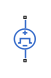# Pulse Voltage Source

Periodic square wave voltage source

•Libraries:
Simscape / Electrical / Sources
Simscape / Electrical / Additional Components / SPICE Sources

## Description

The Pulse Voltage Source block represents a voltage source whose output voltage value is a periodic square pulse as a function of time and is independent of the current through the source. The following equations describe the output voltage as a function of time:

`${V}_{out}\left(0\right)={V}_{1}$`

`${V}_{out}\left(TD\right)={V}_{1}$`

`${V}_{out}\left(TD+TR\right)={V}_{2}$`

`${V}_{out}\left(TD+TR+PW\right)={V}_{2}$`

`${V}_{out}\left(TD+TR+PW+TF\right)={V}_{1}$`

`${V}_{out}\left(TD+PER\right)={V}_{1}$`

where:

• V1 is the output voltage at time zero.

• V2 is the output voltage when the output is high.

• TD is the time at which the pulse first starts.

• TR is the time that it takes the output voltage to rise from V1 to V2.

• TF is the time that it takes the output voltage to fall from V2 to V1.

• PW is the time width of the output pulse.

• PER is the period of the output pulse.

The block determines the values at intermediate time points by linear interpolation.

The specified values for PW and PER have the following effect on the block output:

• If both PW and PER are infinite, the block produces a step response at time TD.

• If PER is infinite and PW is finite, the block produces a single pulse of width PW and infinite period.

• If PW is infinite and PER is finite, the block produces a step response with pulses of width TR to a value V1 every PER seconds.

• If PW > PER, the block produces a step response with pulses of width TR to a value V1 every PER seconds.

## Ports

### Conserving

expand all

Electrical conserving port associated with the pulse voltage source positive voltage

Electrical conserving port associated with the pulse voltage source negative voltage

## Parameters

expand all

Value of the output voltage at time zero, in V.

Value of the output voltage when the output is high, in V.

Time at which the pulse first starts, in s.

Time it takes the output voltage to rise from the Initial value, V1 value to the Pulse value, V2 value. The value must be greater than or equal to `0`.

Time it takes the output voltage to fall from the Pulse value, V2 value to the Initial value, V1 value. The value must be greater than or equal to `0`.

Time width of the output pulse. The value must be greater than `0`.

Period of the output pulse. For the default value, `Inf``s`, the block produces a single pulse with an infinite period.

## Version History

Introduced in R2008a